# Roux Method Tutorial

The Roux method was invented by Gilles Roux, a Frenchman, in 2003. It has 4 main steps, these being

This method is one of the Big Four methods, consisting of CFOP, Petrus, Roux and ZZ. It is widely used, notably by Kian Mansour and Sean Patrick Villanueva, and it is also arguably the best OH method, despite the prevalence of M slice moves. For speedsolving, the average move count is 48 moves, and for FMC the average move count is 28. These stats are using a more advanced LSE than the one taught in this tutorial.

## First Block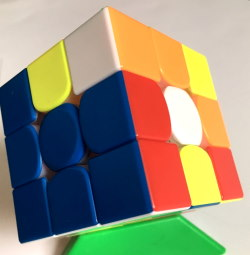This step is the first step in this method.

This step aims to solve a 1x2x3 block on the left, but that can, of course, be rotated to be on the right hand side. This step is usually done by either inserting the DL edge, then solving the two F2L pairs around it, which is usually inefficient, or blockbuilding, which tends to have more moves that are not ergonomic.

Beginners to cubing or to Roux tend to use the former way, but the latter way is better in the long run, and can usually be solved mentally in the 15 second inspection period allowed to you by competition organisers. This step should take about 7 or 8 moves if you always solve it in the same place using the same pieces.

## Second Block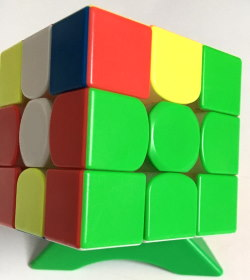Second Block is the second step in this method. It is the same block as the first step, but on the right. This step has more complications than the first step, as you need to solve it without breaking the already solved first block.

This block is occasionally done completely or influenced during the solving of the first block, but that is much harder to do. This block, unlike the initial one, is much easier to do by inserting the DR edge and then solving the pairs.

M moves can be used, as nothing has been solved in that slice yet.

## CMLL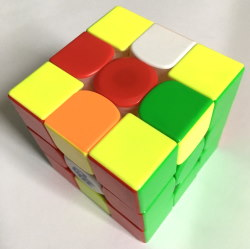CMLL is a step that is pretty similar to COLL, but with one key difference: it does not care if the M slice is solved. This means that algorithms can be shorter. However, it is possible to use COLL algorithms for CMLL, but not the other way around. There are 42 algorithms, and it is recommended that you learn all of them, but you can also just use sune and anti sune to orient the corners, then use Jb and Y perms to position them.

Sune - R U R' U R U2 R'

Antisune - R U2 R' U' R U' R'

Jb Perm - R U R' F' R U R' U' R' F R2 U' R'

Y Perm - F R U' R' U' R U R' F' R U R' U' R' F R F'

### CMLL algorithms

There are seven groups of algorithms, and these are called O, H, Pi, U, T, S, As, and L.

All O group cases have all the corners pre-oriented, all H and Pi group cases have no corners oriented; in H they are arranged in two sets of headlights, one facing away and the other facing towards you, and in Pi they are arranged with a set of headlights facing left or right and the other two corners facing away from each other. All U, T, and L cases have 2 corners oriented, and all S and As cases have only one corner oriented.

#### O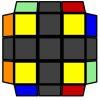R U R' F' R U R' U' R' F R2 U' R'

Diagonal Swap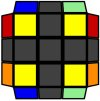F R U' R' U' R U R' F' R U R' U' R' F R F'

#### H

Columns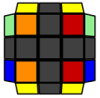R U2 R' U' R U R' U' R U' R'

Rows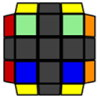F R U R' U' R U R' U' R U R' U' F'
Column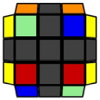(U) R U2' R2' F R F' U2 R' F R F'
Row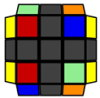(U2) r U' r2' D' r U' r' D r2 U r'

#### Pi

Right Bar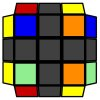F R U R' U' R U R' U' F'

Back Slash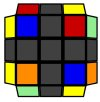(U) F R' F' R U2 R U' R' U R U2' R'

X Checkerboard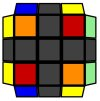(U') R' F R U F U' R U R' U' F'

Forward SlashR U2 R' U' R U R' U2' R' F R F'

Columns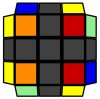(U') r U' r2' D' r U r' D r2 U r'

Left Bar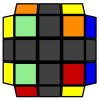(U') R' U' R' F R F' R U' R' U2 R

#### U

Forward Slash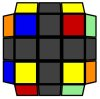(U2) R2 D R' U2 R D' R' U2 R'

Back Slash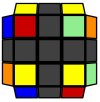R2' D' R U2 R' D R U2 R

Front Row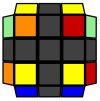R2' F U' F U F2 R2 U' R' F R

Rows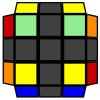(U') F R2 D R' U R D' R2' U' F'

X Checkerboard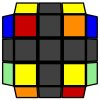(U2) r U' r' U r' D' r U' r' D r

Back Row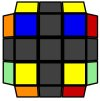(U') F R U R' U' F'

#### T

Left Bar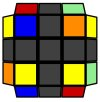(U') R U R' U' R' F R F'

Right Bar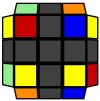(U) L' U' L U L F' L' F

Rows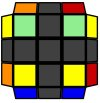F R' F R2 U' R' U' R U R' F2

Front Row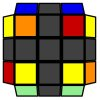r' U r U2' R2' F R F' R

Back Row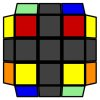r' D' r U r' D r U' r U r'

Columns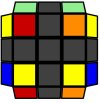r2' D' r U r' D r2 U' r' U' r

#### S

Left Bar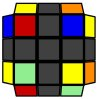(U) R U R' U R U2 R'

X Checkerboard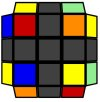(U) L' U2 L U2' L F' L' F

Forward Slash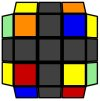(U) F R' F' R U2 R U2' R'

Columns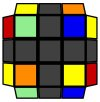(U) R U R' U' R' F R F' R U R' U R U2' R'

Right Bar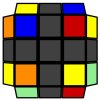R U R' U R' F R F' R U2' R'

Back Slash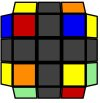(U) R U' L' U R' U' L

#### As

Right Bar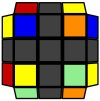(U) R' U' R U' R' U2' R

Columns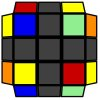(U') R2 D R' U R D' R' U R' U' R U' R'

Back Slash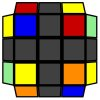(U') F' L F L' U2' L' U2 L

X Checkerboard(U') R U2' R' U2 R' F R F'

Forward Slash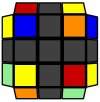(U') L' U R U' L U R'

Left Bar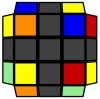R' U' R U' R' U R' F R F' U R

#### L

Mirror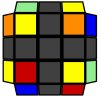(U2) F R U' R' U' R U R' F'

Inverse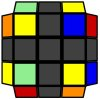(U2) F R' F' R U R U' R'

Pure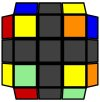R U2 R' U' R U R' U' R U R' U' R U' R'

Front Commutator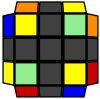(U2) R U2 R D R' U2 R D' R2'

Diagonal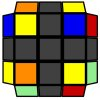R' U' R U R' F' R U R' U' R' F R2

Back Commutator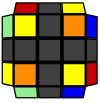(U) R' U2 R' D' R U2 R' D R2

## LSE

LSE, L6E, or Last 6 Edges is the final step in the Roux method. This step has no algorithms, and is done intuitively in one of a few ways.

The original Roux proposal was to orient all the centers and edges, then to permute the UR and UL edges then finally permute the M slice edges. The way that is probably easiest for beginners is to solve the F and B centers, then insert the DB and DF edges, then orient all the U layer edges, then permute them. It is quite inefficient though.

The F and B centers can be solved in a M, M', or M2 move. After this, the DF and DB edges can be inserted by M' U2 M, M U2 M', M' U/U' M or M U/U' M. If the edge is already inserted in one of the slots, take it out by inserting a different edge into there by doing one of the above triggers.

After the above, orienting the U layer is pretty easy. You can either stick to only M and U moves, or you can add in R and r moves to make it faster and more efficient. I will explain both ways.

### MU only

If there are no edges oriented, then do M' U' M U2 M' U' M to turn it into the case where two adjacent edges are oriented.

If there are two opposite edges oriented, then hold them in UF and UB and do
M' U' M U M' U2 M U' M' U2 M U M' U' M to turn it into the case where there are two adjacent edges oriented.

If there are two adjacent edges oriented, then rotate or do U moves until the two oriented edges are in UL and UF. Then, do M' U' M U2 M' U' M to orient all of them.

### MURr moves

If there are two adjacent edges oriented, hold them in UL and UB and do r U R' U' M U R U' R' to orient all the edges.

If there are two opposite edges oriented, hold them in UB and UF and do R U R' U' M' U R U' r' to orient all the edges.

If there are no edges oriented, do the algorithm for one of the above cases, then follow the step for whichever one you end up with.

### Permuting the U layer

There are 4 possibilities when you get to this stage: a Ua perm, a Ub perm, a Z perm, and a H perm.

Ua - M2 U M U2 M' U M2

Ub - M2 U' M U2 M' U' M2

Z - M2 U M2 U M' U2 M2 U2 M'

H - M2 U' M2 U2 M2 U' M2

Congratulations, you have successfully solved the Rubik's Cube with the Roux method.

Article by J8Cubes. CMLL algorithms and case names from Kian Mansour's CMLL list.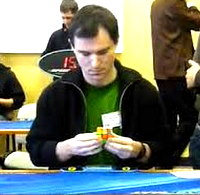Gilles Roux